Demo problem: Large-displacement post-buckling of a pressure-loaded thin-walled elastic ring – displacement-control techniques

In this example we study a more challenging beam problem: The non-axisymmetric buckling of a thin-walled elastic ring, loaded by a spatially-constant external pressure,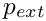. For sufficiently small positive (or arbitrarily large negative) values ofthe ring deforms axisymmetrically and in this mode it is very stiff, implying that large changes in pressure are required to change its radius. However, ifexceeds a critical threshold, the axisymmetric configuration becomes unstable, causing the ring to buckle non-axisymmetrically. Once buckled, the ring is much more flexible and small changes inare sufficient to induce dramatic changes in its shape.

The rapid change in stiffness following the buckling makes it difficult to compute strongly buckled solutions by continuation methods as the solution computed for a certain value ofmay represent a poor approximation of the solution at a slightly larger pressure. In extreme cases, this can cause the Newton method (whose convergence relies on the provision of good initial guesses for the unknowns) to diverge.

We will demonstrate the use of so-called "displacement control" techniques to overcome these problems. Displacement-control techniques are useful in problems in which some a-priori knowledge about the expected displacement field allows us to re-formulate the problem. Rather than prescribing the load on the elastic solid and computing the displacement field, we prescribe the displacement of a carefully-selected material "control" point and regard the load required to achieve this displacement as an unknown.

 Non-axisymmetric buckling of a pressure-loaded, thin-walled elastic ring We wish to compute the deformation of a linearly-elastic, circular ring of undeformed radiusand wall thickness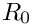, subject to a spatially-constant external pressure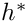. We choose the undeformed radiusas the lengthscale for the non-dimensionalisation of the problem and assume that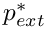, justifying the use of beam theory. As discussed in the previous example we scale the pressure on the ring's effective Young's modulus,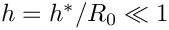, where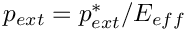. We use the non-dimensional arclength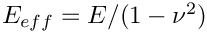along the ring's undeformed centreline as the Lagrangian coordinate and parametrise the position vector to the ring's undeformed centreline as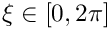We wish to compute the position vector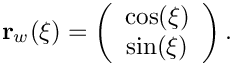to the deformed ring's centreline. Here is a sketch of the problem: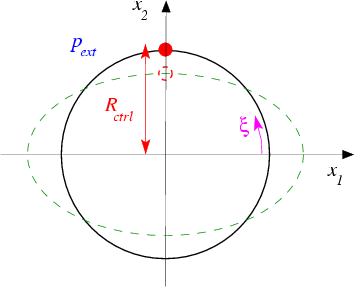Sketch of the buckling ring. Note that we have chosen the Eulerian coordinate axes so that they coincide with the ring's lines of symmetry.

# The expected deformation and the displacement-control formulation of the problem

Standard linear stability analysis [see, e.g., G.J. Simitses "An introduction to the elastic stability of structures", Prentice-Hall, (1976)] predicts the ring to become unstable to non-axisymmetric perturbations with an azimuthal wavenumber of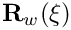at a pressure of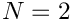For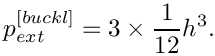we therefore expect the ring to deform into a shape similar to the one indicated by the dashed line in the above sketch. As the ring buckles non-axisymmetrically, the material point on the vertical symmetry line (i.e. the material point with Lagrangian coordinate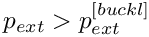) moves radially inwards. This makes it an excellent control point for this problem as we can "sweep" through the entire range of the ring's post-buckling deformation by varying its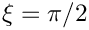– coordinate from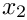(corresponding to the undeformed, axisymmetric configuration) to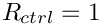(corresponding to a configuration in which the ring is collapsed to the point of opposite wall contact). We note that Flaherty, Keller & Rubinow's analysis [SIAM J. Appl. Math 23, 446–455 (1972)], based on an inextensible beam model, predicts opposite wall contact to occur at an external pressure of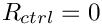To apply displacement control we change the formulation of the problem from

 Original formulation of the problem Determine the position vector to the centreline of the deformed ring,, in terms of the Lagragian coordinate, for a given value of the external pressure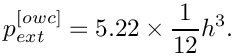.

to

 Displacement-control formulation of the problem Determine the position vector to the centreline of the deformed ring,, in terms of the Lagragian coordinate, for an external pressure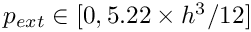that is such that the vertical coordinate of the control point is equal to, i.e.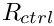where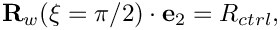is given.

# Results

The figure below shows computed ring shapes for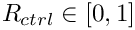. They were obtained in two phases: During the first phase of the computation we subjected the ring to a load of the form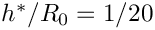where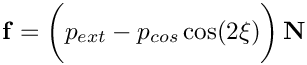is the outer unit normal on the deformed ring and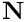is a small cosinusoidal perturbation to the external pressure which forces the ring to buckle "in the right direction". The undeformed configuration was used as the initial guess for an initial computation with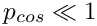[or, in the case of displacement control,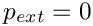]. Subsequently we increased[or decreased] in small steps, using the previously computed solutions for the displacement field [and] as initial guesses for the unknowns. This procedure was continued until the ring was collapsed up to the point of opposite wall contact. During the second phase, we setand reversed the procedure to re-trace the deformation to the axisymmetric state.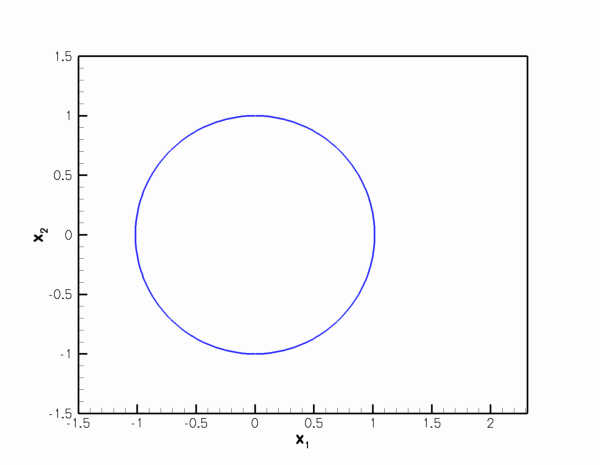Computed ring shapes.

The figure below illustrates the load/displacement characteristics computed by this procedure. The graph shows the radii of two material points on the ring: The green line shows the radius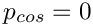of the control point; the red line the radius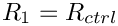of the material point located at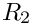, i.e. the material point located on the ring's second line of symmetry. (Because of the symmetry of the buckling pattern this line may also be interpreted as the load-displacement curve for the control point when the ring buckles in the "opposite" direction). The dash-dotted blue line shows the load/displacement curve when the ring deforms axisymmetrically. In this mode, the radii of the two material points are obviously identical. Finally, the dashed lines shows the load/displacement path when the ring is subjected to a non-zero perturbation pressure,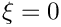, which deforms it non-axisymmetrically so that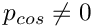even for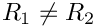.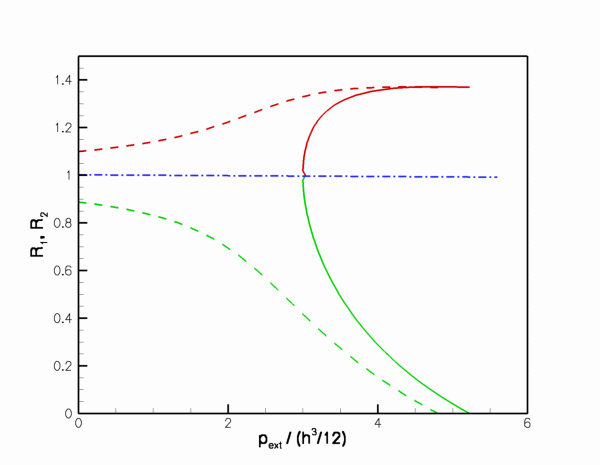Bifurcation diagram for a buckling ring.

The diagram clearly illustrates the enormous change in stiffness when the ring changes from the axisymmetric to the non-axisymmetric regime. The non-axisymmetric regime emanates from the axisymmetric state (via a supercritical bifurcation at a pressure of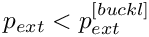, as predicted by the linear stability analysis) and opposite wall contact occurs at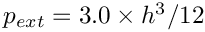, in perfect agreement with Flaherty, Keller & Rubinow's theoretical analysis.

# Applying displacement control in oomph-lib

To facilitate the solution of solid mechanics problems with displacement-control techniques, oomph-lib provides a DisplacementControlElement, which may be used to add the displacement control constraint to the global system of equations that is solved by Newton's method. Applying displacement control in a solid mechanics problem involves the following straightforward steps:

1. Formulate the solid mechanics problems in its "standard form":

In this formulation the (scalar) load level is prescribed and the displacements are regarded as unknowns.

2. Change the representation of the (scalar) load level to allow it to be an unknown in the Problem:

In the original formulation, the load level is likely to have been represented by a double precision number. To allow the load level to be regarded as an unknown, it must be represented by a value in a Data object. Currently, oomph-lib's DisplacementControlElement expects the load level to be the one-and-only value in the load Data object. We note that computations with a prescribed load are still possible and simply require pinning of the value that represents the load.

3. "Tell" the solid mechanics elements that the load level is a (potential) unknown:

Since the load on the solid mechanics elements affects their residuals, their elemental Jacobian matrices (which contain the derivatives of the entries in the elemental residual vector with respect to all unknowns that affect it) must take the dependence on the (potentially) unknown load level into account. This may be achieved by adding the Data object that stores the load level to the element's external Data (i.e. Data whose values affect the element's residual vector, but whose values are not determined by the element). External Data is automatically included in an element's equation numbering procedure. Furthermore, since the elemental Jacobian matrices of SolidFiniteElements are generated by finite-differencing, the derivatives of the element's residual vector with respect to the load level are computed automatically. Consequently, the application of displacement control does not require a re-implementation of the solid mechanics elements.

4. Identify a material point in the solid that can serve as a "control point":

Ideally, this control point should move monotonically along one of the (Eulerian) coordinate directions as the deformation of the solid body increases.

5. Create a DisplacementControlElement and add it to the Mesh.

The DisplacementControlElement adds the displacement constraint to the global system of equations and thus provides the additional equation required to determine the unknown load level. We note that the DisplacementControlElement has two constructors:

• The first version expects a pointer to the Data object whose one-and-only value contains the unknown load level. This version of the constructor is appropriate in cases where the load Data has already been created elsewhere (as described above).

• The second version of the constructor creates the required load Data object internally and provides access to it via a pointer-based access function.

The section "Internal" and "external" Data in elements and "global" Data in Problems provides a more detailed discussion of when to use which version of the constructor.

6. Done!

Set the desired value of the control displacement and solve the problem.

The driver code discussed below illustrates these steps.

# Global parameters and functions

The namespace Global_Physical_Variables, used to define the problem parameters and the load function, is very similar to that used in the previous example without displacement control. They main difference is that the adjustable load (the external pressure) is now defined as a Data value, rather than a double precision number. This allows it to become an unknown in the problem when displacement control is used.

//=======start_of_namespace=========================
/// Namespace for physical parameters
//==================================================
{
/// Nondim thickness
double H=0.05;
/// Prescribed position (only used for displacement control)
double Xprescr = 1.0;
/// Perturbation pressure
double Pcos=0.0;
/// Pointer to pressure load (stored in Data so it can
/// become an unknown in the problem when displacement control is used
Data* Pext_data_pt;
/// Load function: Constant external pressure with cos variation to
/// induce buckling in n=2 mode
const Vector<double> &x,
const Vector<double>& N,
{
for(unsigned i=0;i<2;i++)
{
}
}
/// Return a reference to the external pressure
/// load on the elastic ring.
/// A reference is obtained by de-referencing the pointer to the
/// data value that contains the external load
double &external_pressure()
{return *Pext_data_pt->value_pt(0);}
} // end of namespace
Namespace for physical parameters.
double Xprescr
Prescribed position (only used for displacement control)
void press_load(const Vector< double > &xi, const Vector< double > &x, const Vector< double > &N, Vector< double > &load)
Load function: Constant external pressure with cos variation to induce buckling in n=2 mode.
Data * Pext_data_pt
Pointer to pressure load (stored in Data so it can become an unknown in the problem when displacement...
double Pcos
Perturbation pressure.
double & external_pressure()
Return a reference to the external pressure load on the elastic ring. A reference is obtained by de-r...
double H
Nondim thickness.

# The driver code

The main function builds two different versions of the problem, demonstrating the use of displacement control in the cases when the Data object that contains the adjustable load already exists, or has to be created by the DisplacementControlElement, respectively. The two versions only differ in the The constructor.

//=======start_of_main=================================================
/// Driver for ring test problem
//=====================================================================
int main()
{
// Number of elements
unsigned n_element = 13;
// Displacement control?
bool displ_control=true;
// Label for output
DocInfo doc_info;
// Demonstrate how to use displacement control with already existing load Data
//----------------------------------------------------------------------------
{
//Set up the problem
// Output directory
doc_info.set_directory("RESLT_global");
// Do static run
problem.parameter_study(doc_info);
}
// Demonstrate how to use displacement control without existing load Data
//-----------------------------------------------------------------------
{
//Set up the problem
// Output directory
doc_info.set_directory("RESLT_no_global");
// Reset counter
doc_info.number()=0;
// Do static run
problem.parameter_study(doc_info);
}
} // end of main
////////////////////////////////////////////////////////////////// //////////////////////////////////...
int main()
///////////////////////////////////////////////////////////////////// ///////////////////////////////...

# The problem class

The problem class is very similar to the one used
in the previous example without displacement control. Here we store the number of solid mechanics elements in the Problem's private member data since we will add the DisplacementControlElement to the mesh.

//========start_of_problem_class========================================
/// Ring problem
//======================================================================
template<class ELEMENT>
class ElasticRingProblem : public Problem
{
public:
/// Constructor: Number of elements and flags for displ control
/// and displacement control with existing data respectively.
ElasticRingProblem(const unsigned &n_element,
bool& displ_control,
/// Access function for the specific mesh
OneDLagrangianMesh<ELEMENT>* mesh_pt()
{
return dynamic_cast<OneDLagrangianMesh<ELEMENT>*>(Problem::mesh_pt());
}
/// Update function is empty
/// Update function is empty
/// Doc solution
void doc_solution(DocInfo& doc_info, ofstream& trace_file);
/// Perform the parameter study
void parameter_study(DocInfo& doc_info);
private:
/// Use displacement control?
/// Pointer to geometric object that represents the undeformed shape
GeomObject* Undef_geom_pt;
/// Number of elements in the beam mesh
unsigned Nbeam_element;
}; // end of problem class
GeomObject * Undef_geom_pt
Pointer to geometric object that represents the undeformed shape.
OneDLagrangianMesh< ELEMENT > * mesh_pt()
Access function for the specific mesh.
Constructor: Number of elements and flags for displ control and displacement control with existing da...
void actions_after_newton_solve()
Update function is empty.
void actions_before_newton_solve()
Update function is empty.
bool Displ_control
Use displacement control?
unsigned Nbeam_element
Number of elements in the beam mesh.
void doc_solution(DocInfo &doc_info, ofstream &trace_file)
Doc solution.
void parameter_study(DocInfo &doc_info)
Perform the parameter study.

# The constructor

The first half of the constructor is similar to the one used in the previous example without displacement control. We create a GeomObject (an Ellipse with unit half axes) to define the ring's undeformed geometry, and build the 1D Lagrangian mesh. Note that, because of the symmetry of the buckling pattern, we only discretise one quarter of the domain.

//======start_of_constructor============================================
/// Constructor for elastic ring problem
//======================================================================
template<class ELEMENT>
Displ_control(displ_control),Nbeam_element(n_element)
{
// Undeformed beam is an elliptical ring
Undef_geom_pt=new Ellipse(1.0,1.0);
// Length of the doamin (in terms of the Lagrangian coordinates)
double length=2.0*atan(1.0);
//Now create the (Lagrangian!) mesh
Problem::mesh_pt() =
new OneDLagrangianMesh<ELEMENT>(n_element,length,Undef_geom_pt);

Next we apply the symmetry boundary conditions: Zero vertical [horizontal] displacements and infinite [zero] slope at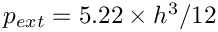[at]. (See the previous example for a more detailed discussion of the boundary conditions for beam elements.)

// Boundary condition:
// Bottom:
unsigned ibound=0;
// No vertical displacement
mesh_pt()->boundary_node_pt(ibound,0)->pin_position(1);
// Infinite slope: Pin type 1 (slope) dof for displacement direction 0
mesh_pt()->boundary_node_pt(ibound,0)->pin_position(1,0);
// Top:
ibound=1;
// No horizontal displacement
mesh_pt()->boundary_node_pt(ibound,0)->pin_position(0);
// Zero slope: Pin type 1 (slope) dof for displacement direction 1
mesh_pt()->boundary_node_pt(ibound,0)->pin_position(1,1);

We now distinguish between the cases with and without displacement control:

• Case 1: No displacement control

If we don't use displacement control, we create the Data object whose one-and-only value stores the adjustable load level (the external pressure), and store the pointer to the newly created Data object in Global_Physical_Variables::Pext_data_pt. Since the value of the external pressure is prescribed (i.e. not an unknown in the problem), we pin the value.

//===========================
if (!Displ_control)
{
// Create Data object whose one-and-only value contains the
//Pin the external pressure because it isn't actually adjustable.

• Case 2: Displacement control

If displacement control is used, we identify the control point (the material point at the "end" of the last element in the mesh) and the (Eulerian) coordinate direction (the vertical coordinate) in which its position is to be controlled:

// Displacement control
//=====================
else
{
// Choose element in which displacement control is applied: the last one
SolidFiniteElement* controlled_element_pt=
dynamic_cast<ELEMENT*>(mesh_pt()->element_pt(Nbeam_element-1));
// Fix the displacement in the vertical (1) direction...
unsigned controlled_direction=1;
//... at right end of the control element
Vector<double> s_displ_control(1);
s_displ_control=1.0;
// Pointer to displacement control element
DisplacementControlElement* displ_control_el_pt;

• Case 2a: Load Data does not yet exist

We can now call the constructor for the DisplacementControlElement:

// Build displacement control element
displ_control_el_pt=
new DisplacementControlElement(controlled_element_pt,
s_displ_control,
controlled_direction,

This version of the constructor creates the load Data object whose one-and-only value stores the adjustable load. We obtain a pointer to the newly-created Data object from the access function DisplacementControlElement::displacement_control_load_pt() and store it in Global_Physical_Variables::Pext_data_pt to make it accessible to the load function Global_Physical_Variables::press_load(...)

// The constructor of the DisplacementControlElement has created
// a new Data object whose one-and-only value contains the

• Case 2b: The load Data already exists

In some applications, the Data object that specifies the adjustable load level might already have been created elsewhere in the Problem. For such cases, oomph-lib provides an alternative constructor whose argument list includes the pointer to the already-existing load Data object. To demonstrate its use we create a suitable Data object and store it in the Problem's "global" Data (see "Internal" and "external" Data in elements and "global" Data in Problems for a more detailed discussion of this step) and pass it to the constructor:

// Demonstrate use of displacement control with some existing data
//----------------------------------------------------------------
else
{
// Create Data object whose one-and-only value contains the
// Currently, nobody's "in charge of" this Data so it won't
// get included in any equation numbering schemes etc.
// --> declare it to be "global Data" for the Problem
// so the Problem is in charge and will perform such tasks.
// Build displacement control element and pass pointer to the
displ_control_el_pt=
new DisplacementControlElement(controlled_element_pt,
s_displ_control,
controlled_direction,
}

In both cases, we add the newly created DisplacementControlElement to the mesh to ensure that it is included in the Problem.

// Add the displacement-control element to the mesh
}

Next, we execute the usual loop over the elements to pass the pointers to the problem's non-dimensional parameters the elements. If displacement control is used, we also pass the pointer to the load Data object to the elements' external Data to indicate that the element's residual vectors depends on the unknown load. Finally, we set up the equation numbering scheme.

//Loop over the elements to set physical parameters etc.
for(unsigned i=0;i<Nbeam_element;i++)
{
//Cast to proper element type
ELEMENT *elem_pt = dynamic_cast<ELEMENT*>(mesh_pt()->element_pt(i));
// Set wall thickness
elem_pt->h_pt() = &Global_Physical_Variables::H;
// Function that specifies load Vector
//Assign the undeformed beam shape
elem_pt->undeformed_beam_pt() = Undef_geom_pt;
// Displacement control? If so, the load on *all* elements
// is affected by an unknown -- the external pressure, stored
// as the one-and-only value in a Data object: Add it to the
// elements' external Data.
if (Displ_control)
{
//The external pressure is external data for all elements
}
}
// Do equation numbering
cout << "# of dofs " << assign_eqn_numbers() << std::endl;
} // end of constructor

# Post-processing

The post-processing function documents the ring shapes and adds the load/displacement characteristics of the two material points on the ring's symmetry lines to the trace file.

//=======start_of_doc=====================================================
/// Document solution
//========================================================================
template<class ELEMENT>
ofstream& trace_file)
{
ofstream some_file;
char filename;
// Number of plot points
unsigned npts=5;
// Output solution
sprintf(filename,"%s/ring%i.dat",doc_info.directory().c_str(),
doc_info.number());
some_file.open(filename);
mesh_pt()->output(some_file,npts);
some_file.close();
// Local coordinates of plot points at left and right end of domain
Vector<double> s_left(1);
s_left=-1.0;
Vector<double> s_right(1);
s_right=1.0;
// Write trace file: Pressure, two radii
trace_file
<< " "
<< dynamic_cast<ELEMENT*>(mesh_pt()->
element_pt(0))->interpolated_x(s_left,0)
<< " "
<< dynamic_cast<ELEMENT*>
(mesh_pt()->element_pt(Nbeam_element-1))->interpolated_x(s_right,1)
<< std::endl;
} // end of doc

# The parameter study

We start by opening a trace file to record the load/displacement characteristics, and output the initial configuration.

//========start_of_run=====================================================
/// Solver loop to perform parameter study
//=========================================================================
template<class ELEMENT>
{
//Open a trace file
char filename;
sprintf(filename,"%s/trace.dat",doc_info.directory().c_str());
ofstream trace_file(filename);
trace_file << "VARIABLES=\"p_e_x_t\",\"R_1\",\"R_2\"" << std::endl;
trace_file << "ZONE" << std::endl;
//Output initial data
doc_solution(doc_info,trace_file);

Next we set up the increment of the control parameter, choosing the displacement or load increments such that the ring's deformation is increased from the axisymmetric initial state to total collapse with opposite wall contact in 11 steps.

// Number of steps
unsigned nstep= 11; //51;
// Increments
double displ_increment=1.0/double(nstep-1);
double p_buckl=3.00*pow(Global_Physical_Variables::H,3)/12.0;
double p_owc =5.22*pow(Global_Physical_Variables::H,3)/12.0;
double pext_increment=(p_owc-p_buckl)/double(nstep-1);
// Set initial values for parameters that are to be incremented
Global_Physical_Variables::Pext_data_pt->set_value(0,p_buckl-pext_increment);

Without displacement-control the Newton method can require a large number of iterations, therefore we increment the maximum number of iterations.

// Without displacement control the Newton method converges very slowly
// as we approach the axisymmetric state: Allow more iterations before
// calling it a day...
if (Displ_control)
{
Max_newton_iterations=100;
}

We start the parameter study by increasing the ring's compression (either by increasing the external pressure or by reducing the- coordinate of the control point) withto induce buckling in the desired direction.

// Downward loop over parameter incrementation with pcos>0
//--------------------------------------------------------
/// Perturbation pressure
// Downward loop over parameter incrementation
//---------------------------------------------
for(unsigned i=1;i<=nstep;i++)
{
// Displacement control?
if (!Displ_control)
{
// Increment pressure
}
else
{
// Increment control displacement
}
// Solve
newton_solve();
// Doc solution
doc_info.number()++;
doc_solution(doc_info,trace_file);
} // end of downward loop

Then we reset the perturbation pressure to zero and reduce the ring's collapse by decreasing the external pressure (or by increasing the- coordinate of the control point).

// Reset perturbation pressure
//----------------------------
// Set initial values for parameters that are to be incremented
// Start new zone for tecplot
trace_file << "ZONE" << std::endl;
// Upward loop over parameter incrementation
//------------------------------------------
for(unsigned i=nstep;i<2*nstep;i++)
{
// Displacement control?
if (!Displ_control)
{
// Increment pressure
}
else
{
// Increment control displacement
}
// Solve
newton_solve();
// Doc solution
doc_info.number()++;
doc_solution(doc_info,trace_file);
}
} // end of run

Done!

## "Internal" and "external" Data in elements and "global" Data in Problems

In the section The constructor we encountered two different constructors for the DisplacementControlElement.

• The first version (in which the Data object that contains the unknown load value is created by the constructor) is most natural for the problem considered here as the load Data is created specifically for the purpose of allowing displacement control to be applied. It therefore natural to regard the DisplacementControlElement as being "in charge of" the load Data , and storing it in the element's "internal Data". (Recall that once Data is stored in an element's internal Data, the element becomes responsible for performing tasks such as equation numbering, timestepping, etc. that must be performed exactly once for each Data value in the Problem.)

• The second version of the constructor is appropriate for problems in which the load Data has already been created by another element, implying that the other element is already "in charge" of the Data object and performs the equation numbering, timestepping etc. for its values. In that case, the load Data is regarded as "external Data" for the DisplacementControlElement.

In our example code, we simulated the second scenario by creating the load Data object before calling the second version of the constructor. While this ensures that the load can be regarded as an unknown in the problem, the Problem remains "unaware" of the additional unknown, as none of the elements in the Problem's mesh is "in charge" of it. While this is clearly a somewhat artificial scenario, oomph-lib provides a mechanism for handling such cases: Adding a Data object to the Problem's "global Data" by calling

puts the Problem itself "in charge" of this Data.

## Exercises

1. Run the code without displacement control (e.g. by setting the boolean flag displ_control in the main function to false) and confirm that a non-zero perturbation pressure,is required to induce the ring's non-axisymmetric collapse This shows that the numerical model is not as sensitive to non-axisymmetric perturbations as the theory suggests – roundoff error alone is not sufficient to initiate non-axisymmetric buckling. Use this version of the code to compute the load-displacement characteristics of the ring in its axisymmetric state, i.e. the dash-dotted blue line in the bifurcation diagram shown at the beginning of this document.

2. Run the code without displacement control and explain why during the second phase of the parameter study (when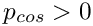), the Newton method converges very slowly and provides very inaccurate results when.

3. We claimed that the load/displacement curves for the post-buckling regime emanate from the axisymmetric branch at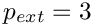, yet closer inspection of the bifurcation diagram shows a small kink in the post-buckling curves near the bifurcation. Explain what causes this kink and why it is practically impossible to avoid its occurrence. [Hint: The load/displacement curves contain individual data points, each one of which corresponds to a solution of the governing equations. The solutions were obtained by Newton's method which tends to converge to a solution in which the unknowns are "close" to their values at the beginning of the iteration. Is it obvious that an initial guess that corresponds to a non-axisymmetric configuration is necessarily "closer" to another non-axisymmetric solution than to a nearby axisymmetric solution?]

4. The computation shown above was performed with the default non-dimensional wall thickness of. Repeat the computation with smaller wall-thicknesses (the previous example shows how to change the default value) and confirm the theoretical predictions for the dependence ofandon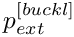.

5. Comment out the command that stores the load Data as global Data and explain why the code fails with a segmentation fault. Use the Problem::self_test() function to identify the problem. [Hint: What is the default value for a Data object's global equation numbers? What happens if this default is not overwritten? Why is the default assignment not overwritten?]